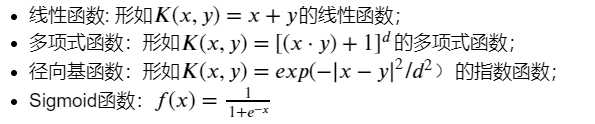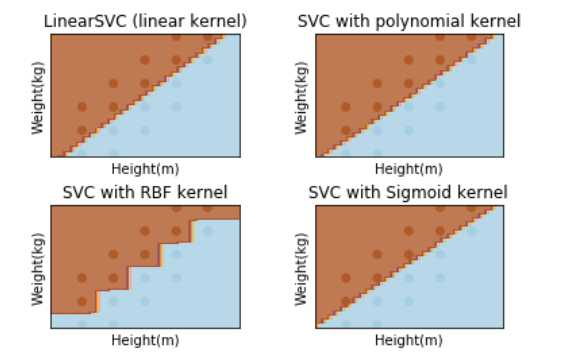# SVM的sklearn.svm.SVC()函数应用

sklearn.svm.SVC(C=1.0,?kernel=‘rbf‘,?degree=3,?gamma=‘auto‘,?coef0=0.0,?shrinking=True,?probability=False,

Tol=0.001,?cache_size200,?class_weight=None,?verbose=False,? max_iter=-1,?

decision_function_shape=None,random_state=None)

? ?

1、C：C-SVC的惩罚参数C默认值是1.0，C越大，相当于惩罚松弛变量，希望松弛变量接近0，即对误分类的惩罚增大，趋向于对训练集全分对的情况，这样对训练集测试时准确率很高，但泛化能力弱。C值小，对误分类的惩罚减小，允许容错，将他们当成噪声点，泛化能力较强。

C一般可以选择为：0.0001 到10000,选择的越大，表示对错误例惩罚程度越大，可能会导致模型过拟合

2、kernel?：核函数，默认是rbf，可以是‘linear‘, ‘poly‘, ‘rbf‘, ‘sigmoid‘, ‘precomputed‘?

? 　　0 – 线性：u‘v

?　　 1 – 多项式：(gamma*u‘*v + coef0)^degree

? 　　2 – RBF函数：exp(-gamma|u-v|^2)

? 　　3 –sigmoid：tanh(gamma*u‘*v + coef0)3、degree?：多项式poly函数的维度，默认是3，选择其他核函数时会被忽略。建议设置为2；

4、gamma?：‘rbf‘，‘poly‘和‘sigmoid‘的核系数。当前默认值为‘auto‘，它使用1 / n_features，如果gamma=‘scale‘传递，则使用1 /（n_features * X.std（））作为gamma的值。当前默认的gamma‘‘auto‘将在版本0.22中更改为‘scale‘。

5、?coef0?：核函数的常数项。对于‘poly‘和 ‘sigmoid‘有用。

6、probability?：默认False。是否启用概率估计。必须在调用fit之前启用它，并且会减慢该方法的速度。

7、shrinking?：默认为true，是否采用shrinking heuristic（收缩启发式）方法

8、?tol?：默认为1e-3，停止训练的误差值大小，

9、cache_size?：默认为200，核函数cache缓存大小

10、?class_weight?：{dict，‘balanced‘}。将类i的参数C设置为SVC的class_weight [i] * C.?如果没有给出，所有类都应该有一个权重。"平衡"模式使用y的值自动调整与输入数据中的类频率成反比的权重n_samples?/?(n_classes?*?np.bincount(y))

11、verbose?：默认False。启用详细输出。请注意，此设置利用libsvm中的每进程运行时设置，如果启用，则可能无法在多线程上下文中正常运行。

12、max_iter?：最大迭代次数。-1为无限制。

13、decision_function_shape?：‘ovo‘, ‘ovr‘ or None, default=None3

14、random_state?：默认 无。伪随机数生成器的种子在对数据进行混洗以用于概率估计时使用。如果是int，则random_state是随机数生成器使用的种子;?如果是RandomState实例，则random_state是随机数生成器;?如果没有，随机数生成器所使用的RandomState实例np.random。

? ?

1）对于线性核函数，没有专门需要设置的参数

2）对于多项式核函数，有三个参数。-d用来设置多项式核函数的最高此项次数，也就是公式中的d，默认值是3。-g用来设置核函数中的gamma参数设置，也就是公式中的第一个r(gamma)，默认值是1/k（k是类别数）。-r用来设置核函数中的coef0，也就是公式中的第二个r，默认值是0。

3）对于RBF核函数，有一个参数。-g用来设置核函数中的gamma参数设置，也就是公式中的第一个r(gamma)，默认值是1/k（k是类别数）。

4）对于sigmoid核函数，两个参数g以及r：gamma一般可选1 2 3 4，coef0选0.2 0.4 0.60.8 1

? ?

support_?：支持向量索引。

support_vectors_?：支持向量。

n_support_?：每一类的支持向量数目

dual_coef_?：决策函数中支持向量的系数

coef_?：赋予特征的权重（原始问题中的系数）。这仅适用于线性内核。

intercept_?：决策函数中的常量。

?

? ?

? ?

---实验练习

? ?

 1 2 3 4 5 6 7 8 9 10 11 12 13 14 15 16 17 18 19 20 21 22 23 24 25 26 27 28 29 30 31 32 33 34 35 36 37 38 39 40 41 42 43 44 45 46 47 48 49 50 51 52 53 54 55 56 57 58 59 60 61 62 63 64 65 66 67 68 69 70 71 %matplotlib inline import numpy as np import scipy as sp from sklearn import svm from sklearn.model_selection import train_test_split # 注意: 如果sklearn是0.18之前的版本，则执行下面的语句： # from sklearn.cross_validation import train_test_split import matplotlib.pyplot as plt #导入数据 data = [] labels = [] # 读取身高体重数据集 with open("./Input/data.txt") as ifile: for line in ifile: tokens = line.strip().split(‘ ‘) # data列表存储身高体重数据 data.append([float(tk) for tk in tokens[:-1]]) # label列表存储此人被定义为胖还是瘦 labels.append(tokens[-1]) # x: 以array形式存储身高体重构成的坐标 x = np.array(data) # labels: 将label列表array化 labels = np.array(labels) y = np.zeros(labels.shape) # y：存储0,1化的体重标签，值为0说明此人被定义为瘦，值为1说明此人定义为胖 y[labels==‘fat‘]=1 x_train, x_test, y_train, y_test = train_test_split(x, y, test_size = 0.0) # 建立画布（可以不用关心具体的实现过程） h = .02 x_min, x_max = x_train[:, 0].min() - 0.1, x_train[:, 0].max() + 0.1 y_min, y_max = x_train[:, 1].min() - 1, x_train[:, 1].max() + 1 xx, yy = np.meshgrid(np.arange(x_min, x_max, h), np.arange(y_min, y_max, h)) # 绘图名称 titles = [‘LinearSVC (linear kernel)‘, ‘SVC with polynomial kernel‘, ‘SVC with RBF kernel‘, ‘SVC with Sigmoid kernel‘] ? ?‘‘‘ svm.SVC(kernel=‘linear‘)：核函数为线性函数 svm.SVC(kernel=‘poly‘, degree=3)：核函数为3次多项式函数，如果degree=n，则使用的核函数是n次多项式函数 svm.SVC()：核函数为径向基函数 svm.SVC(kernel=‘sigmoid‘)：核函数为Sigmoid函数 ‘‘‘ #以下是参数的重点部分： # 核函数为线性函数 clf_linear = svm.SVC(kernel=‘linear‘,C=50).fit(x, y) # 核函数为多项式函数 clf_poly = svm.SVC(kernel=‘poly‘, degree=2,coef0=10).fit(x, y) # 核函数为径向基函数 clf_rbf = svm.SVC(kernel=‘rbf‘,C=100,gamma=0.5).fit(x, y) # 核函数为Sigmoid函数 clf_sigmoid = svm.SVC(kernel=‘sigmoid‘,C=1000000,gamma=0.0001,coef0=-1.5).fit(x, y) ? ?for i, clf in enumerate((clf_linear, clf_poly, clf_rbf, clf_sigmoid)): answer = clf.predict(np.c_[xx.ravel(), yy.ravel()]) plt.subplot(2, 2, i + 1) plt.subplots_adjust(wspace=0.4, hspace=0.4) # 将数据点绘制在坐标图上 z = answer.reshape(xx.shape) plt.contourf(xx, yy, z, cmap=plt.cm.Paired, alpha=0.8) ? plt.scatter(x_train[:, 0], x_train[:, 1], c=y_train, cmap=plt.cm.Paired) plt.xlabel(‘Height(m)‘) plt.ylabel(‘Weight(kg)‘) plt.xlim(xx.min(), xx.max()) plt.ylim(yy.min(), yy.max()) plt.xticks(()) plt.yticks(()) plt.title(titles[i]) plt.show()

? ?? ?

? ?

? ?

? ?

? ?

? ?

? ?

? ?

? ?

? ?

? ?

? ?

? ?

? ?

SVM的sklearn.svm.SVC()函数应用

(0)
(0)